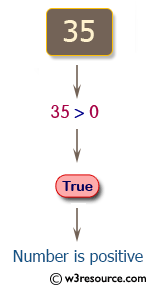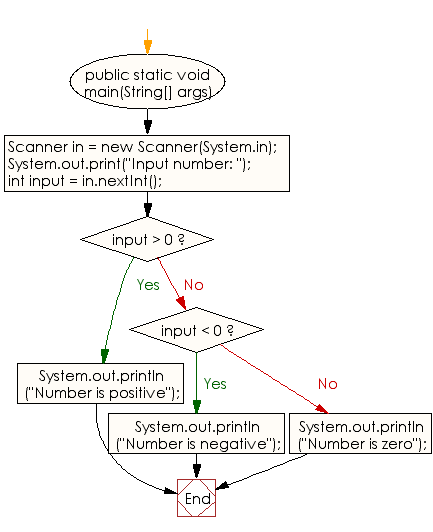﻿ Java - Get a number and check it is positive or negative

# Java: Get a number from the user and print whether it is positive or negative number

## Java Conditional Statement: Exercise-1 with Solution

Write a Java program to get a number from the user and print whether it is positive or negative.

Test Data
Input number: 35

Pictorial Presentation:Sample Solution:

Java Code:

``````import java.util.Scanner;
public class Exercise1 {

public static void main(String[] args)
{
Scanner in = new Scanner(System.in);
System.out.print("Input number: ");
int input = in.nextInt();

if (input > 0)
{
System.out.println("Number is positive");
}
else if (input < 0)
{
System.out.println("Number is negative");
}
else
{
System.out.println("Number is zero");
}
}
}
```
```

Sample Output:

```Input number: 35
Number is positive
```

Flowchart:Java Code Editor:

Improve this sample solution and post your code through Disqus

What is the difficulty level of this exercise?

Test your Programming skills with w3resource's quiz.

﻿

## Java: Tips of the Day

GenerateRandomInt

Generate a random integer between Integer.MIN_VALUE and Integer.MAX_VALUE.

```public static int generateRandomInt() {